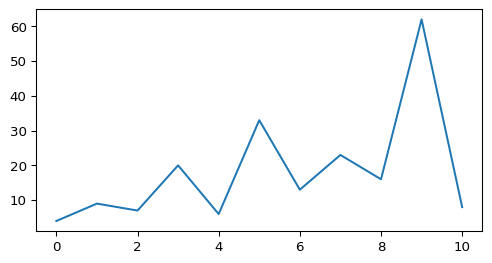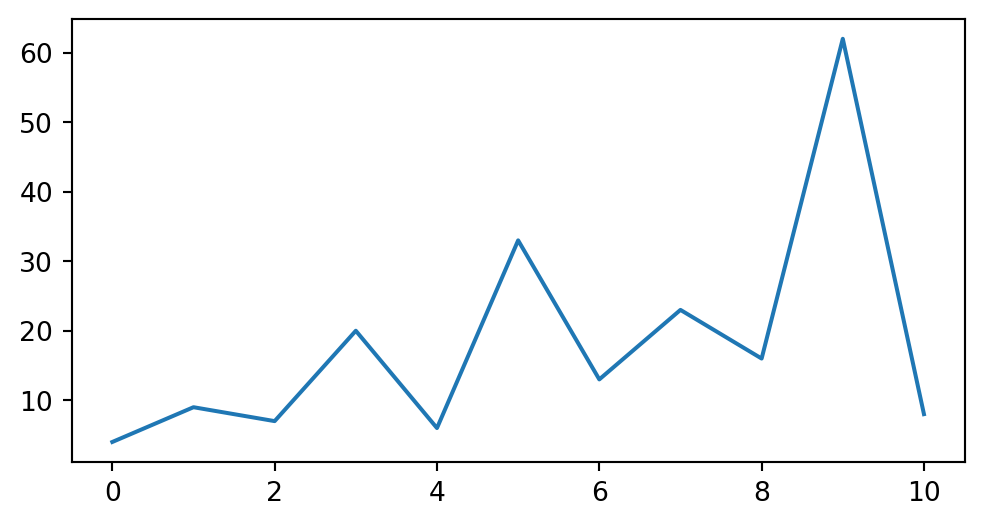# Code Cells#

## Code, Output, Streams#

An empty code cell:

[ ]:




Two empty lines:

[ ]:




:

# 2 empty lines before, 1 after


A simple output:

:

6 * 7

:

42


The standard output stream:

:

print('Hello, world!')

Hello, world!


Normal output + standard output

:

print('Hello, world!')
6 * 7

Hello, world!

:

42


The standard error stream is highlighted and displayed just below the code cell. The standard output stream comes afterwards (with no special highlighting). Finally, the “normal” output is displayed.

:

import sys

print("I'll appear on the standard error stream", file=sys.stderr)
print("I'll appear on the standard output stream")
"I'm the 'normal' output"

I'll appear on the standard output stream

I'll appear on the standard error stream

:

"I'm the 'normal' output"


Note

Using the IPython kernel, the order is actually mixed up, see https://github.com/ipython/ipykernel/issues/280.

## Special Display Formats#

### Local Image Files#

:

from IPython.display import Image
i = Image(filename='images/notebook_icon.png')
i

::

display(i):

from IPython.display import SVG
SVG(filename='images/python_logo.svg')

:### Image URLs#

:

Image(url='https://www.python.org/static/img/python-logo-large.png')

::

Image(url='https://www.python.org/static/img/python-logo-large.png', embed=True)

::

Image(url='https://jupyter.org/assets/homepage/main-logo.svg')

:### Math#

:

from IPython.display import Math
eq = Math(r'\int\limits_{-\infty}^\infty f(x) \delta(x - x_0) dx = f(x_0)')
eq

:

$\displaystyle \int\limits_{-\infty}^\infty f(x) \delta(x - x_0) dx = f(x_0)$
:

display(eq)

$\displaystyle \int\limits_{-\infty}^\infty f(x) \delta(x - x_0) dx = f(x_0)$
:

from IPython.display import Latex
Latex(r'This is a \LaTeX{} equation: $a^2 + b^2 = c^2$')

:

This is a \LaTeX{} equation: $a^2 + b^2 = c^2$
:

%%latex
\begin{equation}
\int\limits_{-\infty}^\infty f(x) \delta(x - x_0) dx = f(x_0)
\end{equation}

\begin{equation} \int\limits_{-\infty}^\infty f(x) \delta(x - x_0) dx = f(x_0) \end{equation}

### Plots#

Make sure to use at least version 0.1.6 of the matplotlib-inline package (which is an automatic dependency of the ipython package).

By default, the plots created with the “inline” backend have the wrong size. More specifically, PNG plots (the default) will be slightly larger than SVG and PDF plots.

This can be fixed easily by creating a file named matplotlibrc (in the directory where your Jupyter notebooks live, e.g. in this directory: matplotlibrc) and adding the following line:

figure.dpi: 96


If you are using Git to manage your files, don’t forget to commit this local configuration file to your repository. Different directories can have different local configurations. If a given configuration should apply to multiple directories, symbolic links can be created in each directory.

For more details, see Default Values for Matplotlib’s “inline” Backend.

By default, plots are generated in the PNG format. In most cases, it looks better if SVG plots are used for HTML output and PDF plots are used for LaTeX/PDF. This can be achieved by setting nbsphinx_execute_arguments in your conf.py file like this:

nbsphinx_execute_arguments = [
"--InlineBackend.figure_formats={'svg', 'pdf'}",
]


In the following example, nbsphinx should use an SVG image in the HTML output and a PDF image for LaTeX/PDF output (other Jupyter clients like JupyterLab will still show the default PNG format).

:

import matplotlib.pyplot as plt

:

fig, ax = plt.subplots(figsize=[6, 3])
ax.plot([4, 9, 7, 20, 6, 33, 13, 23, 16, 62, 8]);For comparison, this is how it would look in PNG format …

:

%config InlineBackend.figure_formats = ['png']

:

fig

:… and in 'png2x' (a.k.a. 'retina') format:

:

%config InlineBackend.figure_formats = ['png2x']

:

fig

:### Pandas Dataframes#

Pandas dataframes should be displayed as nicely formatted HTML tables (if you are using HTML output).

:

import numpy as np
import pandas as pd

:

df = pd.DataFrame(np.random.randint(0, 100, size=[10, 4]),
columns=[r'$\alpha$', r'$\beta$', r'$\gamma$', r'$\delta$'])
df

:

$\alpha$ $\beta$ $\gamma$ $\delta$
0 67 38 25 26
1 85 25 22 29
2 41 63 44 65
3 54 60 10 88
4 69 40 86 94
5 30 86 96 26
6 36 30 95 5
7 65 46 15 35
8 95 86 39 13
9 61 5 63 94

### Markdown Content#

:

from IPython.display import Markdown

:

md = Markdown("""
# Markdown

It *should* show up as **formatted** text
with things like [links] and images.

![Jupyter notebook icon](images/notebook_icon.png)

## Markdown Extensions

There might also be mathematical equations like
$a^2 + b^2 = c^2$
and even tables:

A     | B     | A and B
------|-------|--------
False | False | False
True  | False | False
False | True  | False
True  | True  | True

""")
md

:


#### Markdown#

It should show up as formatted text with things like links and images.##### Markdown Extensions#

There might also be mathematical equations like $$a^2 + b^2 = c^2$$ and even tables:

A

B

A and B

False

False

False

True

False

False

False

True

False

True

True

True

:

from IPython.display import YouTubeVideo

:


### Interactive Widgets (HTML only)#

The basic widget infrastructure is provided by the ipywidgets module. More advanced widgets are available in separate packages, see for example https://jupyter.org/widgets.

The JavaScript code which is needed to display Jupyter widgets is loaded automatically (using RequireJS). If you want to use non-default URLs or local files, you can use the nbsphinx_widgets_path and nbsphinx_requirejs_path settings.

:

import ipywidgets as w

:

slider = w.IntSlider()
slider.value = 42
slider

:


A widget typically consists of a so-called “model” and a “view” into that model.

If you display a widget multiple times, all instances act as a “view” into the same “model”. That means that their state is synchronized. You can move either one of these sliders to try this out:

:

slider

:


You can also link different widgets.

Widgets can be linked via the kernel (which of course only works while a kernel is running) or directly in the client (which even works in the rendered HTML pages).

Widgets can be linked uni- or bi-directionally.

Examples for all 4 combinations are shown here:

:

link = w.IntSlider(description='link')

:

:

tabs = w.Tab()
for idx, obj in enumerate([df, fig, eq, i, md, slider]):
out = w.Output()
with out:
display(obj)
tabs.children += out,
tabs.set_title(idx, obj.__class__.__name__)
tabs

:


Other Languages

The examples shown here are using Python, but the widget technology can also be used with different Jupyter kernels (i.e. with different programming languages).

#### Troubleshooting#

To obtain more information if widgets are not displayed as expected, you will need to look at the error message in the web browser console.

To figure out how to open the web browser console, you may look at the web browser documentation:

The error is most probably linked to the JavaScript files not being loaded or loaded in the wrong order within the HTML file. To analyze the error, you can inspect the HTML file within the web browser (e.g.: right-click on the page and select View Page Source) and look at the <head> section of the page. That section should contain some JavaScript libraries. Those relevant for widgets are:

<!-- require.js is a mandatory dependency for jupyter-widgets -->
<script crossorigin="anonymous" integrity="sha256-Ae2Vz/4ePdIu6ZyI/5ZGsYnb+m0JlOmKPjt6XZ9JJkA=" src="https://cdnjs.cloudflare.com/ajax/libs/require.js/2.3.4/require.min.js"></script>
<!-- jupyter-widgets JavaScript -->
<script type="text/javascript" src="https://unpkg.com/@jupyter-widgets/html-manager@^0.18.0/dist/embed-amd.js"></script>
<!-- JavaScript containing custom Jupyter widgets -->
<script src="../_static/embed-widgets.js"></script>


The two first elements are mandatory. The third one is required only if you designed your own widgets but did not publish them on npm.js.

If those libraries appear in a different order, the widgets won’t be displayed.

Here is a list of possible solutions:

• If the widgets are not displayed, see #519.

• If the widgets are displayed multiple times, see #378.

### Arbitrary JavaScript Output (HTML only)#

:

%%javascript

var text = document.createTextNode("Hello, I was generated with JavaScript!");
// Content appended to "element" will be visible in the output area:
element.appendChild(text);


### Unsupported Output Types#

If a code cell produces data with an unsupported MIME type, the Jupyter Notebook doesn’t generate any output. nbsphinx, however, shows a warning message.

:

display({
'text/x-python': 'print("Hello, world!")',
'text/x-haskell': 'main = putStrLn "Hello, world!"',
}, raw=True)


Data type cannot be displayed: text/x-python, text/x-haskell

## ANSI Colors#

The standard output and standard error streams may contain ANSI escape sequences to change the text and background colors.

:

print('BEWARE: \x1b[1;33;41mugly colors\x1b[m!', file=sys.stderr)
print('AB\x1b[43mCD\x1b[35mEF\x1b[1mGH\x1b[4mIJ\x1b[7m'
'KL\x1b[49mMN\x1b[39mOP\x1b[22mQR\x1b[24mST\x1b[27mUV')

ABCDEFGHIJKLMNOPQRSTUV

BEWARE: ugly colors!


The following code showing the 8 basic ANSI colors is based on https://tldp.org/HOWTO/Bash-Prompt-HOWTO/x329.html. Each of the 8 colors has an “intense” variation, which is used for bold text.

:

text = ' XYZ '
formatstring = '\x1b[{}m' + text + '\x1b[m'

print(' ' * 6 + ' ' * len(text) +
''.join('{:^{}}'.format(bg, len(text)) for bg in range(40, 48)))
for fg in range(30, 38):
for bold in False, True:
fg_code = ('1;' if bold else '') + str(fg)
print(' {:>4} '.format(fg_code) + formatstring.format(fg_code) +
''.join(formatstring.format(fg_code + ';' + str(bg))
for bg in range(40, 48)))

            40   41   42   43   44   45   46   47
30  XYZ  XYZ  XYZ  XYZ  XYZ  XYZ  XYZ  XYZ  XYZ
1;30  XYZ  XYZ  XYZ  XYZ  XYZ  XYZ  XYZ  XYZ  XYZ
31  XYZ  XYZ  XYZ  XYZ  XYZ  XYZ  XYZ  XYZ  XYZ
1;31  XYZ  XYZ  XYZ  XYZ  XYZ  XYZ  XYZ  XYZ  XYZ
32  XYZ  XYZ  XYZ  XYZ  XYZ  XYZ  XYZ  XYZ  XYZ
1;32  XYZ  XYZ  XYZ  XYZ  XYZ  XYZ  XYZ  XYZ  XYZ
33  XYZ  XYZ  XYZ  XYZ  XYZ  XYZ  XYZ  XYZ  XYZ
1;33  XYZ  XYZ  XYZ  XYZ  XYZ  XYZ  XYZ  XYZ  XYZ
34  XYZ  XYZ  XYZ  XYZ  XYZ  XYZ  XYZ  XYZ  XYZ
1;34  XYZ  XYZ  XYZ  XYZ  XYZ  XYZ  XYZ  XYZ  XYZ
35  XYZ  XYZ  XYZ  XYZ  XYZ  XYZ  XYZ  XYZ  XYZ
1;35  XYZ  XYZ  XYZ  XYZ  XYZ  XYZ  XYZ  XYZ  XYZ
36  XYZ  XYZ  XYZ  XYZ  XYZ  XYZ  XYZ  XYZ  XYZ
1;36  XYZ  XYZ  XYZ  XYZ  XYZ  XYZ  XYZ  XYZ  XYZ
37  XYZ  XYZ  XYZ  XYZ  XYZ  XYZ  XYZ  XYZ  XYZ
1;37  XYZ  XYZ  XYZ  XYZ  XYZ  XYZ  XYZ  XYZ  XYZ


ANSI also supports a set of 256 indexed colors. The following code showing all of them is based on http://bitmote.com/index.php?post/2012/11/19/Using-ANSI-Color-Codes-to-Colorize-Your-Bash-Prompt-on-Linux.

:

formatstring = '\x1b[38;5;{0};48;5;{0}mX\x1b[1mX\x1b[m'

print('  + ' + ''.join('{:2}'.format(i) for i in range(36)))
print('  0 ' + ''.join(formatstring.format(i) for i in range(16)))
for i in range(7):
i = i * 36 + 16
print('{:3} '.format(i) + ''.join(formatstring.format(i + j)
for j in range(36) if i + j < 256))

  +  0 1 2 3 4 5 6 7 8 91011121314151617181920212223242526272829303132333435
0 XXXXXXXXXXXXXXXXXXXXXXXXXXXXXXXX
16 XXXXXXXXXXXXXXXXXXXXXXXXXXXXXXXXXXXXXXXXXXXXXXXXXXXXXXXXXXXXXXXXXXXXXXXX
52 XXXXXXXXXXXXXXXXXXXXXXXXXXXXXXXXXXXXXXXXXXXXXXXXXXXXXXXXXXXXXXXXXXXXXXXX
88 XXXXXXXXXXXXXXXXXXXXXXXXXXXXXXXXXXXXXXXXXXXXXXXXXXXXXXXXXXXXXXXXXXXXXXXX
124 XXXXXXXXXXXXXXXXXXXXXXXXXXXXXXXXXXXXXXXXXXXXXXXXXXXXXXXXXXXXXXXXXXXXXXXX
160 XXXXXXXXXXXXXXXXXXXXXXXXXXXXXXXXXXXXXXXXXXXXXXXXXXXXXXXXXXXXXXXXXXXXXXXX
196 XXXXXXXXXXXXXXXXXXXXXXXXXXXXXXXXXXXXXXXXXXXXXXXXXXXXXXXXXXXXXXXXXXXXXXXX
232 XXXXXXXXXXXXXXXXXXXXXXXXXXXXXXXXXXXXXXXXXXXXXXXX


You can even use 24-bit RGB colors:

:

start = 255, 0, 0
end = 0, 0, 255
length = 79
out = []

for i in range(length):
rgb = [start[c] + int(i * (end[c] - start[c]) / length) for c in range(3)]
out.append('\x1b['
'38;2;{rgb};{rgb};{rgb};'
'48;2;{rgb};{rgb};{rgb}mX\x1b[m'.format(rgb=rgb))
print(''.join(out))

XXXXXXXXXXXXXXXXXXXXXXXXXXXXXXXXXXXXXXXXXXXXXXXXXXXXXXXXXXXXXXXXXXXXXXXXXXXXXXX